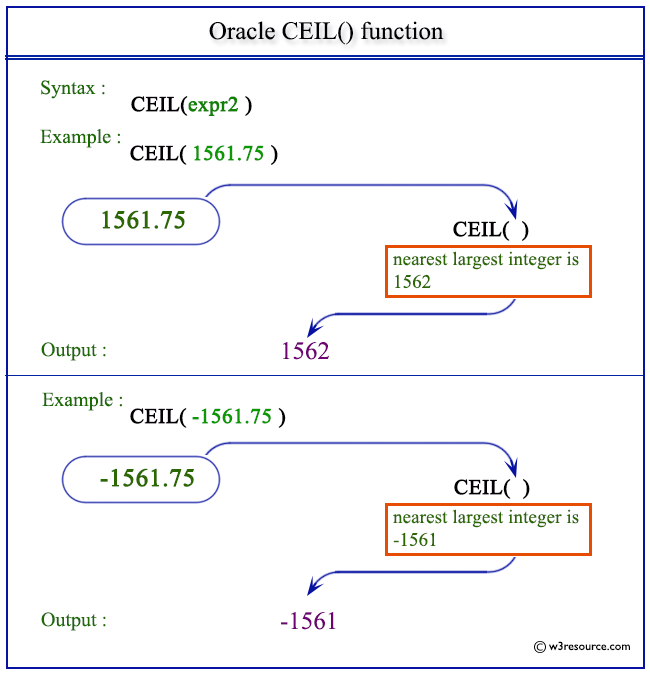# Oracle CEIL() function

## Description

The CEIL() function returns the smallest integer that is not less than the number specified as an argument.
The function takes any numeric or nonnumeric data type (can be implicitly converted to a numeric data type) as an argument.

Syntax:

`CEIL(N);`

Parameters:

Name Description
N A number.

The function returns the same data type as the numeric data type of the argument.

Pictorial Presentation of CEIL() functionExample:

The statement below returns 1562 which is the smallest integer value not less than the value specified (1561.75) in the argument.

``````SELECT 1561.75 AS "Number", CEIL(1561.75)
FROM dual;
```
```

Here is the result

```    Number CEIL(1561.75)
---------- -------------
1561.75          1562
```

Example: CEIL() function with negative value

The statement below returns -1561 which is the smallest integer value not less than the value specified (-1561.75) in the argument.

``````SELECT -1561.75 AS "Number", CEIL(-1561.75)
FROM dual;
```
```

Here is the result

```    Number CEIL(-1561.75)
---------- --------------
-1561.75          -1561
```

Previous: BITAND
Next: COS

﻿